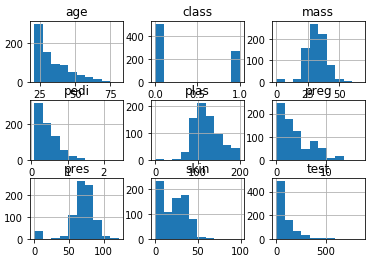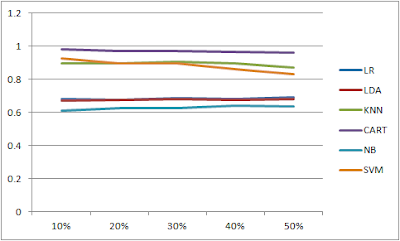## Posts

### Breast Cancer Classification Program

I took another example for Classification problem which is Breast Cancer Diagnostic Data Set. As I have already done 3 examples of Classification program, my objective for this was to go deeper into each line of code. I got to know how to slice an array using NumPy, and also understanding Correlation Matrix. So I'll not again going to explain every step as it follows same steps as done for previous Classification Programs.I did observe different algorithms from a different perspective and I'm sharing these observations in this post. In previous posts, I was looking at accuracy score of different algorithms and then choose the "best" one (having highest accuracy score) to do the validation and check the final statistics.In this example, I tried to check validation output for each algorithm and compare the difference. I've provided the details below which is self-explanatory. However a couple of things I would like to highlight are Confusion Matrix and Classificati…

### Understanding Correlation Matrix PlotI’m loving this top down approach. Initially, I started with completing a ML project without clear understanding of how it is working as expected. Once I got the understanding at 10,000 ft level, I’m diving one step deeper to get into the understanding of “how” and “why”.Today I got into the deeper understanding of Correlation Matrix Plot. I have used this plot in my projects before. However I did not know why I’m executing certain code. In this post, I’ll explain what each line of code is doing.For this exercise, I took a new data set “Breast Cancer Wisconsin (Diagnostic) Data Set”. This data set has 32 attributes, hence a classic match to understand correlation matrix plot.
Before we get into the plot, I need to load the data set. As usual I’ll import necessary libraries and then load the data. Since I have explained these before, I’m not going to repeat and I’ll simply show the code.import numpy as npimport pandas as pdfrom pandas import read_csv# Load dataset
url = "https://arc…

### Array Slicing using NumPy

When I started working on a new classification project, I had to slice data array to get the desired result. That’s when I realized I have not understood array slicing completely. That prompted me to get my hands dirty on array slicing and I’ll share my learning in this post.We need to use numpy library to play around arrays. So I’ll first import numpy.import numpy as npNext, I’ll create a 2-d array with random numbers.arr2d = np.random.randint(10, size=(4, 5))This is how the array looks likearray([[3, 7, 3, 2, 0],
[8, 1, 6, 1, 9],
[3, 3, 3, 8, 2],
[5, 9, 5, 1, 3]])Essentially, there are two parts while describing this array.
arr2d[rowFrom:rowTo-1, columnFrom:columnTo-1]The first section tells numpy how many (or which) rows we are interested in, and the second section tells numpy how many (or which) columns we are interested in.If I don’t mention any values in those sections like this:arr2d[:,:]It shows me the complete array.array([[3, 7, 3, 2, 0],
[8, 1, 6, 1, 9],
[3, 3, 3, 8, 2],
[5, 9, 5,…

### Data Visualization using Pandas - Multivariate PlotsIn the previous post, I explained about data visualization specifically Univariate plots. In this post, I’ll describe Multivariate plots.Multivariate plots shows correlation between multiple variables. I have used the same Pima Indians Diabetes Database.Correlation Matrix Plot
Correlation shows how two variables are related for changes. Let’s take an example. I’ve taken the code as it is from https://machinelearningmastery.com/.# Correction Matrix Plot
import matplotlib.pyplot as plt
import pandas
import numpy
url = "https://raw.githubusercontent.com/jbrownlee/Datasets/master/pima-indians-diabetes.csv"
names = ['preg', 'plas', 'pres', 'skin', 'test', 'mass', 'pedi', 'age', 'class']
correlations = data.corr()
# plot correlation matrix
fig = plt.figure()
cax = ax.matshow(correlations, vmin=-1, vmax=1)
fig.colorbar(cax)
ticks = numpy.arange(0,9,1)
ax.set_x…

### Data Visualization using Pandas - Univariate PlotsData visualization is an important step in ML. That helps us to understand the data in visual representation. Why does it matter? Because it helps us to understand the distribution of the data, any outliers, and also relationship between multiple attributes. Experts also mention that understanding the distribution helps us in choosing correct algorithm. I cannot comment on this statement as of now, as I do not have enough knowledge on that. When I reach that stage, I’ll definitely share.Pandas library provides methods to plot data in multiple ways. In fact, I did use one of those plots called Box and Whisker Plots in my previous examples. However, those plots were used to see the difference between multiple algorithms. In this post, I’ll share how to visualize the data which is an important step much before we apply any ML algorithm.
I’ll use the data set which was used for Binary Classification. The data set is  Pima Indians Diabetes Database which has 8 features.If we plot attributes…

### Binary Classification with PythonIn the previous two examples, I worked on Multi-Class Classification problems, wherein the class (output) can be one of the multiple values.In this example, I took up a Binary Classification problem where the output is either 1 or 0.The approach is similar to previous two examples, so I would only highlight on important points.For the Binary Classification program, the data set taken is Pima Indians Diabetes Database. In this data set, there are 8 features:  1. Number of times pregnant
2. Plasma glucose concentration a 2 hours in an oral glucose tolerance test
3. Diastolic blood pressure (mm Hg)
4. Triceps skin fold thickness (mm)
5. 2-Hour serum insulin (mu U/ml)
6. Body mass index (weight in kg/(height in m)^2)
7. Diabetes pedigree function
8. Age (years)The output is Class variable with values 0 or 1. Class 1 represent tested positive for diabetes. There are 768 rows in this data set.The accuracy for different set of algorithms for this classification looks like this: As can …

### Different Train/Test SplitContinuing with the Car classification  program, I did a small experiment with the splitting of data into Training and Test set. In the previous programs, this split was done with 80:20 ratio. That is 80% of the data was used to train a model and the remaining 20% was used to predict.I wanted to see the difference in accuracy if we change this split ratio. I tested with 4 additional split ratio for test data i.e., 10%, 30%, 40%, and 50%.I verified each of those 6 algorithms for each 5 ratio (including 20%). Here is the interesting result. The y- axis represent the accuracy of the model.
I was expecting a considerable change, but the data doesn’t reflect that. Perhaps, the volume of the data set might not have brought the change I was expecting. Again, I’m not sure about that at this point of time, but i’ll test with a huge volume of data in future.Only the algorithms SVM, KNN, and NB have shown noticeable change from 10% to 50%. The rest of the algorithms have negligible changes.As I s…